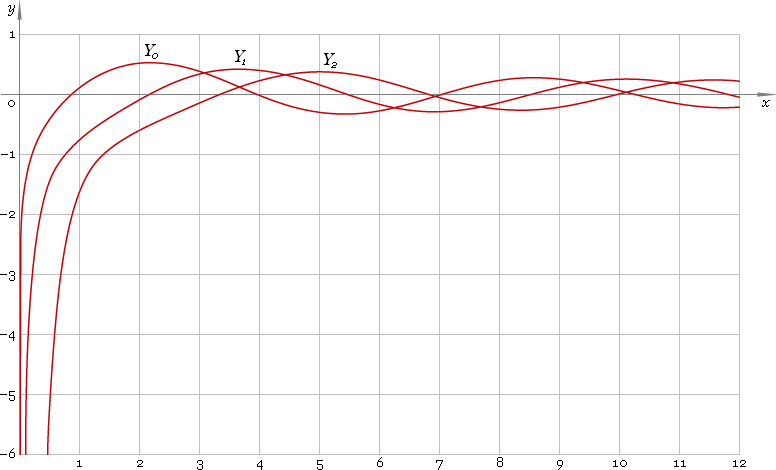The Art of Interface

# Y — Bessel function of the second kind

Category. Mathematics.

Abstract. Bessel function of the second kind of the real (fractional) order: definition, plot, properties and identities.

## 1. Definition

By definition Bessel function is solution of the Besssel equation

z2 w′′ + z w′ + (z2 − ν2) w = 0

As second order equation it has two solutions, second of which has singularity at 0 and is called Bessel function of the second kind — Yν. Parameter ν is called order of the function.

First solution has no singularity at 0 and is called Bessel function of the first kind — Jν.

## 2. Plot

Bessel functions of the second kind defined on positive part of the real axis, at 0 functions have singularity, so, their domain is (0, +∞). Plots of the first three representatives of the second kind Bessel function family depicted below — fig. 1.Fig. 1. Plot of the Bessel functions of the second kind y = Y0(x), y = Y1(x) and y = Y2(x).

## 3. Identities

Next order recurrence:

Yν+1(z) = 2ν /z Yν(z) − Yν−1(z)

Negative argument:

Yν(−z) = eiπν Yν(z) + i 2 cos(πν) Jν(z) = cos(πν) Yν(z) + i [2 cos(πν) Jν(z) − sin(πν) Yν(z)]

For the case of integer order ν=n the negative argument identity can be simplified down to:

Yn(−z) = (−1)n Yn(z) + i (−1)n 2 Jn(z)

and for the case of half-integer order ν=n+1/2 the identity can be simplified down to:

Yn+1/2(−z) = i (−1)n+1 Yn+1/2(z)

Reflection — negative order:

Y−ν(z) = cos(πν) Yν(z) + sin(πν) Jν(z)

For the case of integer order ν=n the reflection identity can be simplified down to:

Y−n(z) = (−1)n Yn(z)

and for the case of half-integer order ν=n+1/2 the identity can be simplified down to:

Y−n−1/2(z) = (−1)n Jn+1/2(z)

## 4. Support

Bessel function of the second kind Yν of the real (fractional) order and complex argument is supported by professional version of the Librow calculator.

## 5. Interface

Bessel function call looks like

``Y(order, argument);``

or

``BesselY(order, argument);``

where order is the function real order, and argument — function argument.

## 6. How to use

To calculate Bessel function of the second kind of the 0 order of the number:

``Y(0, 1.5);``

or:

``BesselY(0, 1.5);``

To calculate Bessel function of the second kind of the 1.2 order of the current result:

``Y(1.2, rslt);``

or:

``BesselY(1.2, rslt);``

To calculate Bessel function of the second kind of the 2.5 order of the number z in memory:

``Y(2.5, mem[z]);``

or:

``BesselY(2.5, mem[z]);``